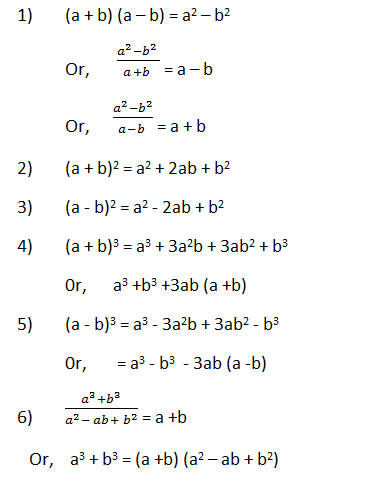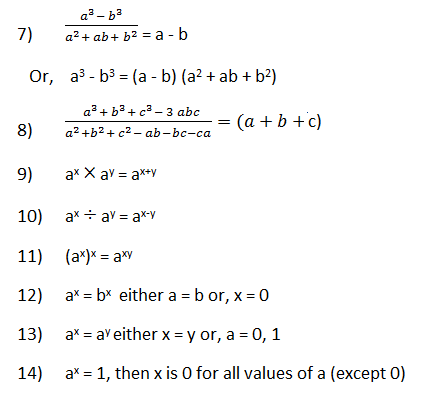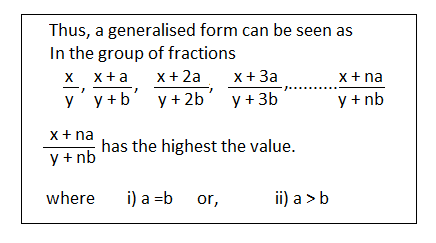# Number System - Concepts and Tricks

If you want to test whether any number is a prime number or not take an integer larger than the approximate square root of that number.

Let it be ‘x’. Test the divisible of the given number by every a prime number less than ‘x’. If it is not divisible by any of them, then it is prime number; otherwise it is a composite number (other than prime).

1. Is 349 a prime number ?
Solution: The square root of 349 is approximate 19. The prime numbers less than 19 are 2, 3, 5, 7, 11, 13, and 17.

Clearly, 349 is not divisible by any of them. Therefore, 349 is a prime number.

2. Is 881 a prime number?
Solution:
The approximate Sq. root of 881 is 30.

Prime number less than 30 is 2, 3, 5, 7, 11, 13, 17, 19, 23, and 29. 881 is not divisible by any of the above numbers, so it is a prime  number.

### RULE OF SIMPLIFICATION

(i) In simplification an expression, first of all vinculum or bar must be removed. For example: we know that(ii) After removing the bar, the brackets are removed, strictly in the order (), {}, [].

(iii)  After removing brackets, we must follow following order of operations :- (a) of (b) division (c) multiplication (d) addition (e) subtraction.

This is also known as the rule of ‘VBODMAS’ , where V, B, O, D, M, A, and S stand for Vinculum, Bracket, Of, Division, Multiplication, Addition and Subtraction respectively.## GENERAL RULE FOR SOLVING PROBLEMS IN ARITHMETIC### Ascending or descending orders in Rational Numbers

#### Rule 1

When the numerator and the denominator of the fractions increase by a constant value, the last fraction is the biggest.
Ques. Which one of the following fractions is the greatest?
3/4,  4/5  and 5/6
Solution:  We see that the numerators as well as denominators of the above fractions increase by 1, so the last fraction, i.e., 5/6, is the greatest fraction.#### Rule 2

The fraction whose numerator after cross – multiplication gives the greater value is greater.

Example: Which is greater: 5/8  or 9/14 ?
Solution:  Step 1:  cross – multiply the two given fractions#### What's trending in BankExamsToday

Smart Prep Kit for Banking Exams by Ramandeep Singh - Download here